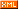# 简单，可复制

:: :: :: :: :: :::: ::431 随笔 :: 0 文章 :: 593 评论 :: 0 引用

# 指针的概念

int *ptr;  char *ptr;  int **ptr;  int (*ptr);  int *(*ptr);

# 指针的类型

int *ptr; //指针的类型是int *  char *ptr; //指针的类型是char *  int **ptr; //指针的类型是 int **  int (*ptr); //指针的类型是 int(*)  int *(*ptr); //指针的类型是 int *(*)

# 指针所指向的类型

int *ptr; //指针所指向的类型是int  char *ptr; //指针所指向的的类型是char  int **ptr; //指针所指向的的类型是 int *  int (*ptr); //指针所指向的的类型是 int()  int *(*ptr); //指针所指向的的类型是 int *()

# 指针的算术运算

 char a;   int *ptr=a;  ...  ...   ptr++;

int array;  int *ptr=array;  ...  //此处略去为整型数组赋值的代码。  ...  for(i=0;i<20;i++)  {      (*ptr)++;      ptr++；  }

char a;  int *ptr = a;  ...  ...  ptr += 5;

# 运算符&和*

int a=12;  int b;  int *p;  int **ptr;  p=&a;//&a的结果是一个指针，类型是int*，指向的类型是int，指向的地址是a的地址。  *p=24;//*p的结果，在这里它的类型是int，它所占用的地址是p所指向的地址，显然，*p就是变量a。ptr=&p;//&p的结果是个指针，该指针的类型是p的类型加个*，在这里是int**。该指针所指向的类型是p的类型，这里是int*。该指针所指向的地址就是指针p自己的地址。 *ptr=&b;//*ptr是个指针，&b的结果也是个指针，且这两个指针的类型和所指向的类型是一样的，所以?amp;b来给*ptr赋值就是毫无问题的了。**ptr=34;//*ptr的结果是ptr所指向的东西，在这里是一个指针，对这个指针再做一次*运算，结果就是一个int类型的变量。

# 指针表达式

int a,b;  int array;  int *pa;  pa=&a;//&a是一个指针表达式。  int **ptr=&pa;//&pa也是一个指针表达式。  *ptr=&b;//*ptr和&b都是指针表达式。  pa=array;  pa++;//这也是指针表达式。

char *arr;  char **parr=arr;//如果把arr看作指针的话，arr也是指针表达式  char *str;  str=*parr;//*parr是指针表达式  str=*(parr+1);//*(parr+1)是指针表达式  str=*(parr+2);//*(parr+2)是指针表达式

# 数组和指针的关系

int array={0,1,2,3,4,5,6,7,8,9},value;  ...  ...  value=array;//也可写成：value=*array;  value=array;//也可写成：value=*(array+3);  value=array;//也可写成：value=*(array+4);

char *str={  "Hello,this is a sample!",  "Hi,good morning.",  "Hello world"  };  char s；  strcpy(s,str);//也可写成strcpy(s,*str);  strcpy(s,str);//也可写成strcpy(s,*(str+1));  strcpy(s,str);//也可写成strcpy(s,*(str+2));

*str也是一个指针，它的类型是char*，它所指向的类型是char，它指向的地址是字符串"Hello,this is a sample!"的第一个字符的地址，即'H'的地址。 str+1也是一个指针，它指向数组的第1号单元，它的类型是char**，它指向的类型是char *。

*(str+1)也是一个指针，它的类型是char*，它所指向的类型是char，它指向"Hi,good morning."的第一个字符'H'，等等。

int array;  int (*ptr);  ptr=&array;

int (*ptr);

sizeof(int(*))==4  sizeof(int )==40  sizeof(ptr)==4

# 指针和结构类型的关系

struct MyStruct  {  int a;  int b;  int c;  }  MyStruct ss={20,30,40};//声明了结构对象ss，并把ss的三个成员初始化为20，30和40。MyStruct *ptr=&ss;//声明了一个指向结构对象ss的指针。它的类型是MyStruct*,它指向的类型是MyStruct。int *pstr=(int*)&ss;//声明了一个指向结构对象ss的指针。但是它的类型和它指向的类型和ptr是不同的。

ptr->a;  ptr->b;  ptr->c;

*pstr；//访问了ss的成员a。  *(pstr+1);//访问了ss的成员b。  *(pstr+2)//访问了ss的成员c。

int array={35,56,37};  int *pa=array;

*pa;//访问了第0号单元  *(pa+1);//访问了第1号单元  *(pa+2);//访问了第2号单元

# 指针和函数的关系

int fun1(char*,int);  int (*pfun1)(char*,int);  pfun1=fun1;  ....  ....  int a=(*pfun1)("abcdefg",7);//通过函数指针调用函数。

posted on 2011-12-13 17:39  ggjucheng  阅读(140475)  评论(26编辑  收藏